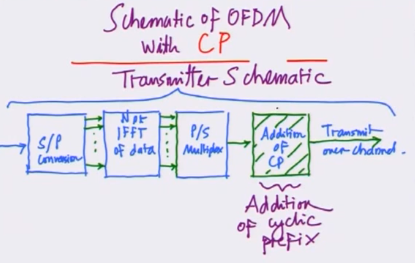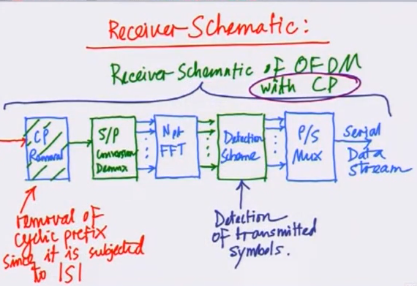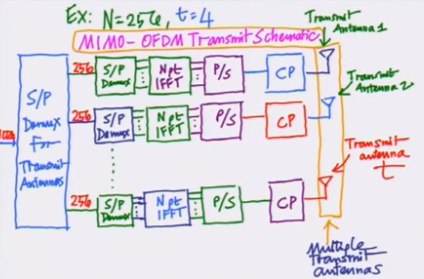# OFDM and SC-FDMA Lectures

## OFDM and multi-carrier modulation

Orthogonal Frequency Division Multiplexing (OFDM) is the basis of LTE. With OFDM, a wideband carrier is transmitted as narrowband sub-carriers. An example is used to compare and contrast single carrier vs. multi-carrier modulation. The symbol time and bandwidth of OFDM sub-carriers is compared with the bandwidth can symbol duration of a single carrier system with the same bandwidth.

This lecture develops a basic mathematical model for a multi-carrier transmission. The detection of a multi-carrier signal is described and analyzed with a simple model.## IFFT sampling for OFDM

This lecture further explores multi-carrier modulation and demodulation. Coherent demodulation of the L-th sub-carrier on an N sub-carrier system is developed from first principles.

Schematic of a multi-carrier modulator and demodulator is explained. We further see how a multi-carrier system with a total bandwidth B and N sub-carriers removes the effects of inter-symbol interference. In a multi-carrier system N symbols of duration N/B are transmitted in parallel. This results in individual carriers having a bandwidth that is much less than the coherence bandwidth in the multi-carrier.

The main issue with multi-carrier systems is that the cost of N modulators and demodulators is prohibitive. This issue is addressed by the ingenious use of the inverse fast Fourier transform as a multi-channel modulator.

## OFDM schematic and cyclic prefix

We look at how OFDM replaces a bank of modulators with an inverse fast Fourier transform. The schematic of an OFDM system is simple:

Serial to parallel conversion of information symbols → IFFT → Parallel to serial conversion of OFDM symbols

In OFDM a Cyclic Prefix (CP) is inserted between OFDM symbols. The CP is formed by inserting the trailing end samples of an OFDM symbol before the OFDM symbol start. This way the leading end of the OFDM symbol is interfering with its own trailing end. This is equivalent to performing a circular convolution between the channel and the symbol (y = h ⊗ x). CP insertion converts a frequency selective channel into a flat fading channel.

An N point DFT recovers the original information symbols. The information symbol transmitted on the k-th subcarrier is recovered with the relation: Y(k) = H(k) x X(k)## OFDM based parallelization and example

This lecture continues the discussion of parallel channel encoding and decoding with OFDM. The role of CP in converting a frequency selective channel into a flat fading channel is covered in detail.

Addition of CP does lower spectral efficiency. The impact of this is minimal for systems with large number of sub-carriers. The CP duration needed is just dependent on the channel delay spread, so it remains constant with increasing sub-carriers. The symbol duration increases with larger N. Thus the percentage of bandwidth wasted due to CP transmission goes down with increasing sub-carrier count.

The OFDM concepts are further illustrated with an example.

## example and introduction to MIMO OFDM

The lecture starts with an example of a fixed profile WiMax with 256 sub-carriers. Each sub-carrier has a bandwidth of 15.625 KHz. This is much less than the coherence bandwidth of 300 KHz. Thus a broadband communication channel has been converted into parallel narrowband channels. In the example, the OFDM symbol duration is 64 microseconds and the cyclic prefix is 8 microseconds.

MIMO OFDM is discussed next. MIMO-OFDM changes a frequency selective MIMO channel to a flat fading MIMO channel. We also observe that the output of a MIMO OFDM system can be modeled as a MIMO FIR filter.

Next, the lecture looks at the MIMO-OFDM transmitter schematic. We note that the IFFT operation and CP insertion takes place at each transmit antenna. MIMO-OFDM receiver is also introduced towards the end of the lecture.## more MIMO OFDM

The lecture looks at the matrix representation of the MIMO. Zero forcing receiver and the MMSE receiver are also covered. Calculation of the channel matrices across all sub-carrier is discussed.

The lecture concludes with an introductory discussion on the impact of a frequency offset in an OFDM system. OFDM inter-carrier orthogonality is lost if the receiver synchronization leaves a uncorrected frequency offset.

## impact of frequency offset on OFDM

We look at the distorting effects of carrier frequency offset, i.e. the transmitter and receiver are not synchronized resulting in a frequency offset in OFDM demodulation. The loss of orthogonality results in inter sub-carrier interference. The signal to interference+noise ratio (SINR) of this inter sub-carrier is modeled.## PAPR in OFDM and introduction to SC-FDMA

Multiple parallel transmissions on sub-carriers result in a high PAPR (Peak to Average Power Ratio). The PAPR increases with increasing sub-carriers. This is captured using a CCDF distribution.

Handling high PAPR places demands on the power amplifier as it requires a larger linear range to maintain distortion free transmission.

The PAPR issue led the LTE designers to choose SC-FDMA in the uplink direction. In SC-FDMA, the transmitter adds an m-size FFT stage before the OFDM transmitter. The m-stage FFT stage takes a single carrier channel and converts it into sub-carrier level symbols that can be transmitted over an N-stage OFDM system (Here m < N)## more SC-FDMA

Introduction of the m-point FFT significantly reduces the PAPR of the system.

The SC-FDMA receiver is discussed next. The receiver performs the N-point FFT to extract the individual sub-carriers. This is followed by equalization and an m-point IFFT to recover the single carrier that was carried over m-sub-carriers in the N-sub-carrier system. After the m-point IFFT we get the single carrier symbols